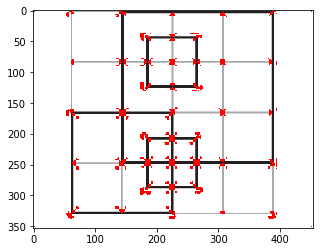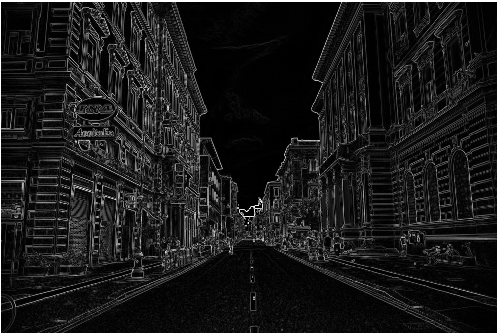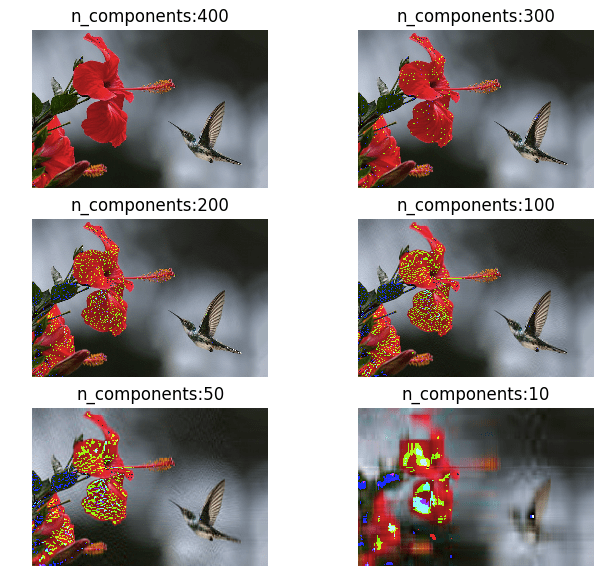## Deskewing scanned documents using horizontal projections

An important pre-processing step in any OCR tool or algorithm is to deskew the scanned document first. Take a look at the below sample scanned image, its tilted by a small angle. In this article I will explain a method to deskew these types of documents using their horizontal projections. The final outcome of deskewing …

## K-fold cross validation

The greatest headache for any machine learning engineer is the problem of overfitting. The model we trained works perfectly on the training dataset but when applied to other new dataset it fails miserably. This is because of overfitting where our classifier learns the provided dataset accurately but fails when applied on new data. One good …

## Draw bounding box around contours – skimage

The basic idea is to find the Xmin, Xmax, Ymin, Ymax of each identified coordinates of the contour and then create a rectangle using it. Lets understand this with an example:   Import the necessary libraries, read the input file, convert it to grayscale and plot it. from skimage.measure import find_contours from skimage.io import imread …## Harris Corner Detector implementation in python

The Harris corner detection algorithm also called the Harris & Stephens corner detector is one of the simplest corner detectors available. The idea is to locate interest points where the surrounding neighbourhood shows edges in more than one direction. The basic idea of algorithm is to find the difference in intensity for a displacement of …

## Linear Regression using Gradient Descent Algorithm

Gradient descent is an optimization method used to find the minimum value of a function by iteratively updating the parameters of the function. Parameters refer to coefficients in Linear Regression and weights in Neural Networks. In a linear regression problem, we find a modal that gives an approximate representation of our dataset. In the below …## Sobel Feldman operator or Sobel filter

Sobel operator is used in computer vision particularly in edge detection algorithms. The operator uses two 3×3 kernels which are convolved with the original image to calculate the image derivatives – one for horizontal changes, and one for vertical. If we define A as the original image, and Gx and Gy are two images which …## Converting Color Images to Grayscale using numpy and some Mathematics

An extremely magnified image at the end is just blocks of colors called pixels, where each pixel is formed by the combination of Red, Blue and Green, our primary colors. RGB color space or RGB color system, constructs all the colors from the combination of the intensities of Red, Green and Blue colors. The red, green and …## Mathematics of Principal component analysis

Principal component analysis is a method used to reduce the number of dimensions in a dataset without losing much information. It’s used in many fields such as face recognition and image compression, and is a common technique for finding patterns in data and also in the visualization of higher dimensional data. PCA is all about …

## Understanding the Classification report through sklearn

A Classification report is used to measure the quality of predictions from a classification algorithm. How many predictions are True and how many are False. More specifically, True Positives, False Positives, True negatives and False Negatives are used to predict the metrics of a classification report as shown below. The report is copied from our previous …

## K-Means on Iris Dataset

Read my previous post to understand how K-Means algorithm works. In this post I will try to run the K-Means on Iris dataset to classify our 3 classes of flowers, Iris setosa, Iris versicolor, Iris virginica (our classess) using the flowers sepal-length, sepal-width, petal-length and petal-width (our features) Getting data: import numpy as np import matplotlib.pyplot …